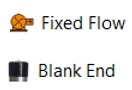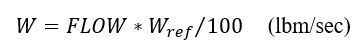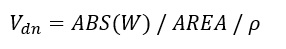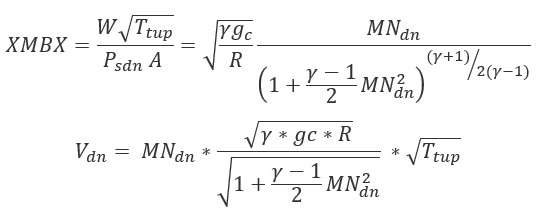# Fixed Flow Elements

## Fixed Flow Element General Description

The Fixed Flow Element is used to supply a constant flow rate either to or from the element/component. Flow is assumed to leave the element at the temperature specified for the upstream chamber. The momentum can be transferred downstream by providing the Exit area, angles, and rotation inputs. When flow is put into a model through a fixed flow element, care must be taken that the temperature in the upstream chamber has any needed correction made for rotational effects to yield the appropriate relative temperature for the downstream chamber. If the downstream chamber is an inertial chamber an absolute temperature must be supplied. If not, consideration must be given to the downstream chamber’s rotational velocity.

## Quick Guide for Fixed Flow Element Creation in the GUIFixed Flow Elements are available under both Compressible Gas Elements & Incompressible Liquid Elements sections. There are five different subtypes of Fixed Flow elements available in Flow Simulator basically differing from the input that are supplied. They are

1. Mass Flow Rate: Value input for mass flow rate.
2. Mass Flow Rate (% Core): Percentage with respect to the Reference Flow Rate (see General Data Section).
3. Volumetric Flow rate: Value input for the Volumetric flow rate.
4. Blank End: The blank end acts as a flow source, but with the flow set to zero.
5. Liquid/Gas Separator: Extract a percentage of the gas or liquid from the upstream chamber.

## Fixed Flow Element Input Variables

Index UI Name (.flo Label) Description
1 Flow Input (SUB_TYPE) Element subtype determines how FLOW variable is interpreted.

1.0: Mass Flow rate is input.

2.0: Mass Flow rate is input as %WREF.

3.0: Volumetric Flow rate is input.

4.0: Blank End with no input.

5.0: Liquid/Gas Separator.

Default value = 1.

2 Flow (FLOW) Element fixed flow rate
3 Exit Area (AREA) Physical area used to calculate exit velocity (Optional input).
4 Element Inlet Orientation: Tangential Angle (THETA) Angle between the element centerline at the entrance of the element and the reference direction.

If the element is rotating or directly connected to one or more rotating elements, the reference direction is defined as parallel to the engine center line and the angle is the projected angle in the tangential direction. Otherwise, the reference direction is arbitrary but assumed to be the same as the reference direction for all other elements attached to the upstream chamber.

Element exit orientation for the theta angle is the same in the inlet THETA for fixed flow element types.

5 Element Inlet Orientation: Radial Angle (PHI) Angle between the element center line at the entrance of the element and the THETA direction. (spherical coordinate system)

Element exit orientation for the PHI angle is the same in the inlet PHI for fixed flow element types.

6 Rotor Index (RPMSEL) Reference rotor index for user-supplied swirl.

Stationary (Database Value = 0.0).

Rotor 1 (Database Value = 1.0): Points to general data Shaft 1 Rotor Speed.

Rotor 2 (Database Value = 2.0): Points to general data Shaft 2 Rotor Speed.

Rotor 3 (Database Value = 3.0): Points to general data Shaft 3 Rotor Speed.

Rotates with Air (database Value = -1.0): Element RPM is based on upstream fluid RPM.

(Do not use zero unless the fixed flow is not rotating).

8 (INCOMPR_FLG)
9 Heat Mode (HEAT_MODE) Mode of heat transfer to/from the fluid in the element.

10 Heat Input (QIN) The value entered for QIN depends on the HEAT_MODE chosen.

11 Phase to Separate (SEP_MODE) This input only used for the SUB_TYPE= Liquid/Gas Separator.
• 0: Remove a fraction of the gas mass from the upstream chamber.
• 1: Remove a fraction of the liquid mass from the upstream chamber.
12 Separation Fraction (0-1) (SEP_FRACT) This input is only used for the SUB_TYPE= Liquid/Gas Separator.

Fraction of the gas or liquid that is removed from the upstream chamber (number between 0.0 and 1.0).

## Fixed Flow Element Theory

If the element is declared to be subtype 1 and subtype 3, the flow going into the downstream chamber is the value input for flow (FLOW). For subtype 2, the flow going into the downstream chamber is:If Exit Area is greater than 0, then

For Incompressible flow:For Compressible Flow:XMBX = Total pressure flow parameter

Nomenclature Subscripts
W: Mass flow rate up: Upstream station
Tt: Total Temperature dn: Downstream station
Pt: Total pressure ref: Reference
Ps: Static pressure
Specific heat Ratio
R: Gas Constant
Density
MN: Vena Contracta Mach Number
gc: Gravitational Constant

## Fixed Flow Element Output Variables

Name Description Units
ELEMENT_RPM RPM (Rotor index)

(Usually an echo of the user input unless modified inside Flow Simulator.)